Exploring working principle of vacuum diode by virtual laboratory | Статья в журнале «Молодой ученый»

Отправьте статью сегодня! Журнал выйдет 1 мая, печатный экземпляр отправим 5 мая.

Опубликовать статью в журнале
Проекты
Меню

Рубрика: Физика

Дата публикации: 12.05.2020

Статья просмотрена: 4 раза

### Библиографическое описание:

Азимов, Сарвар. Exploring working principle of vacuum diode by virtual laboratory / Сарвар Азимов, Ж. Ж. Гуломов, Ш. А. Сатимов, И. М. Мадаминова. — Текст : непосредственный // Молодой ученый. — 2020. — № 19 (309). — С. 89-91. — URL: https://moluch.ru/archive/309/69919/ (дата обращения: 19.04.2021).

﻿

In this article, describe basic theory of working principle of vacuum diode. Besides, give results which are taken by virtual laboratory.

Key words: Vacuum diode, Visual Basic, virtual laboratory, cathode, electron

When it comes to technology, one of the turning points in technology is the vacuum diode. Today, many people think that the vacuum diode has been replaced by a semiconductor diode. But vacuum diodes are still used in space stations. This is because semiconductor diodes are very sensitive to pressure and temperature.

To fully understand the principle of operation of a vacuum diode and the phenomena that take place inside, we need to analyze the phenomenon of thermoemission by applying it to a deeper vacuum diode. Thermoemission is the phenomenon of electrons flying off the surface of a metal when heated. Therefore, as a heated metal, we call the distance between the cathode and the anode equal to x = d and the potential difference between them. Because of this potential difference, electrons move from the cathode to the anode, and electrons move with acceleration toward the anode, and they can be recorded . Electric current flows from the anode to the cathode, and the current density in one direction is [A / m2].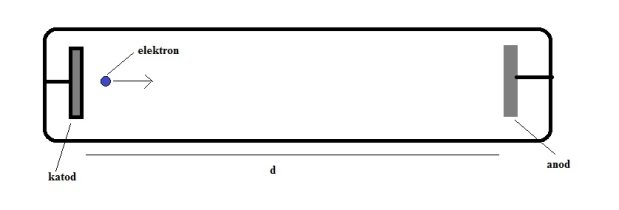Figure 1. Vacuum diode

The current density inside the vacuum diode is as follows: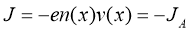(1)

Kinetic energy of electrons: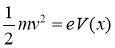(2)

The current density inside the vacuum diode is as follows: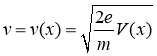(3)

Electrons moving with acceleration produce a current density, and we can see that n (x) decreases and v (x) increases towards the anode.

We want to know how space is related to the charge density and the potential difference between the cathode and the anode. For this we can use the Poisson equation .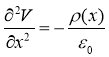(4)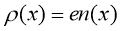(5)

Using formula 1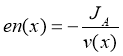(6)

If we combine formulas 6 and 3(7)

If we combine formulas 4 and 7(8)

In the above 8 formulas, the second-order linear differential equation is formed. The solution to this equation is very simple:(9)

This differential equation can be calculated by a simple step-by-step integration.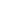The initial conditions at x = 0 are as follows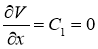(10)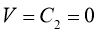(11)(12)(13)

Here, if we say x = d, then V (d) = VA(14)

The above formula 14 represents the relationship between current density and voltage for a Langmur-Child vacuum vacuum diode.

If we increase the current density on the surface, the current is released. That is, the cross-sectional area of the diode lamp is S:(15)

This gives us the theoretical volt-ampere characteristic for a vacuum diode. Unlike a semiconductor diode, a vacuum diode has no reverse current .

One of the demands of the 21st century is the widespread use of these computer technologies in all fields. An example of this is when students use virtual labs directly to understand the nature of laboratories in physics. The advantage of this is that it includes all theories and animations, which helps the student to fully understand the essence of physical processes. Our virtual laboratory, called Vacuum Diode, which we created in the Visual Basic 6.0 programming language, has such a wide range of possibilities. Get a better idea of its interface to do so we have shown its working window in Figure 2.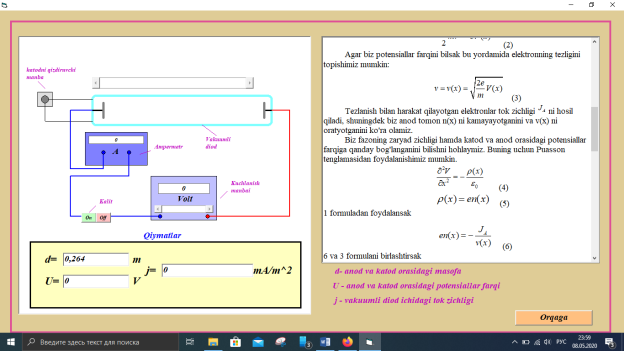Fig. 2. Interface of program

References:

1. Parker, P.: 'Electronics' (Arnold, 1950), pp. 650–657 and 696.
2. Thompson, B. J.: 'Space-Current Flow in Vacuum-TubeStructures', Proceedings of the Institute of Radio Engineers,1943, 31, p. 485.
3. Spenke, E.: 'Electronic Semiconductors' (McGraw-Hill,1958).

## Ключевые слова

Vacuum diode, Visual Basic, virtual laboratory, cathode, electron
Задать вопрос